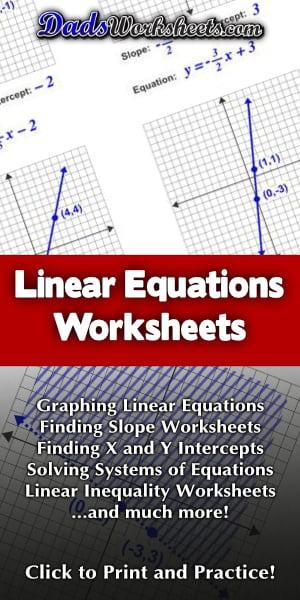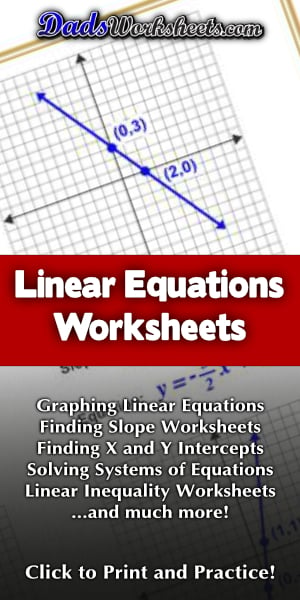One Dad. Four daughters. 9,393 worksheets... and counting!

# Linear Equations

These linear equations worksheets cover graphing equations on the coordinate plane from either y-intercept form or point slope form, as well as finding linear equations from two points. The slope worksheets on this page have exercises where students identify the direction of slope, as well as calculating slope from points on the coordinate plane.

## Graphing Linear Equations

### 8 Linear Equations Worksheets

Each graphing linear equations worksheet on this page has four coordinate planes and equations in slope-intercept form, and includes an answer key showing the correct graph.

## Find the Equation of a Line

### 8 Linear Equations Worksheets

Each linear equations worksheet on this page shows four graphs on a coordinate plane, each with two points labeled, and students find the equation in slope-intercept form by calculating both the slope and y-intercept.

## Point Slope Form

### 8 Linear Equations Worksheets

Each graphing linear equations worksheet on this page has four coordinate planes and equations in point-slope form, and includes an answer key showing the correct graph.

## Graphing Linear Inequalities

### 8 Linear Equations Worksheets

Each graphing linear inequalities worksheet on this page has four coordinate planes and linear inequalities in slope-intercept form, and includes an answer key showing the correct graph.

## Systems of Equations

### 8 Linear Equations Worksheets

The worksheets on this page have four coordinate planes and systems equations in point-slope form that students graph to solve, and includes an answer key showing the correct graph.

## Slope Worksheets

### 12 Linear Equations Worksheets

The slope worksheets on this page require students to identify the direction of a the slope of a line, and also to find the slope from two points.